# Sort Names by Middle Name in Excel

This post will guide you how to sort full name by middle name in excel 2013, or 2016. How do I sort names by middle name with excel formula. How to sort full names by middle name using Text to Columns command in excel.

## Sort Names by Middle Name using Excel Formula

Assuming that you have a list of names in your worksheet and you would like to alphabetize by middle name. You can create an excel formula based on the IF function, the ISERR function, the FIND function, and the MID function.

You need to extract the middle name firstly, then you can use the sort command to sort the middle names.  So we need to create a formula to get the middle name from the full name.

Let’s see the following example to sort full names in range B2:B4 by middle name.

1# you can write down the following formula to get middle name:

`=IF(ISERR(FIND(“ “,B2, FIND(“ “,B2)+1))),” “, MID(B2, FIND(“ “,B2)+1, FIND(“ “,B2, FIND(“ “,B2)+1))-FIND(“ “, B2)-1))`

Or you can use the following formula to get middle name:

`= MID(B2,FIND(" ",B2)+1,FIND("#",SUBSTITUTE(B2," ","#",2),1)- FIND(" ",B2,1)-1)`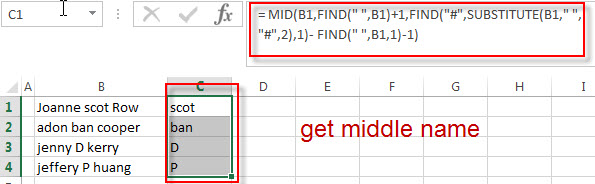If you want to know how this formula works, you can refer to this post: How to get First name, Middle name and Last name.

2# select the cells that contain middle name in range C1:D4, and then click “DATA”-> Sort A to Z.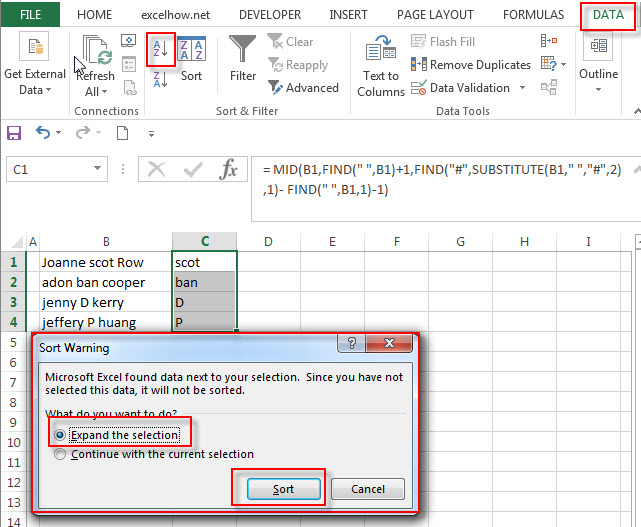3# select “Expand the selection” radio button. Then click “Sort” button. You will see that the full names have been sorted by middle names.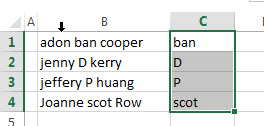## Sort Names by Middle Name using Text to Columns command

You can also use the Text to Columns command to extract the middle name from a full name in excel. Then you can sort the full name by middle name cell. Just refer to the following steps:

1# Select the range of cells that contains full name, then click on Data -> Text to Columns (under Data Tools group)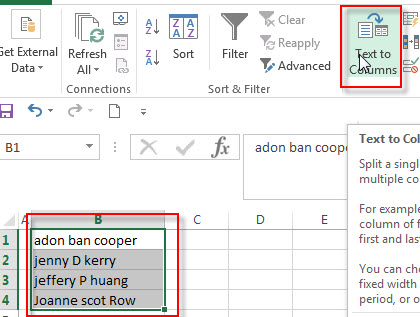2# the “Convert Text to Columns Wizard” windows will appear. Then select Delimited radio button. Click “Next” button.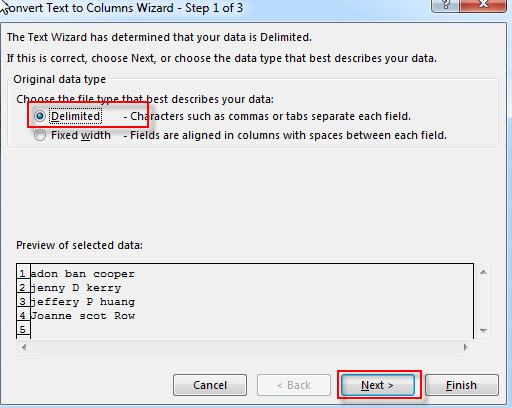3# select Space option in Delimiters section box, then click “Next” button.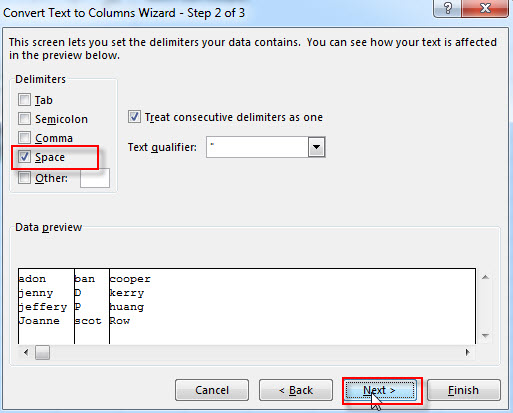4# choose Destination Cell, such as: C1, then click “Finish” button.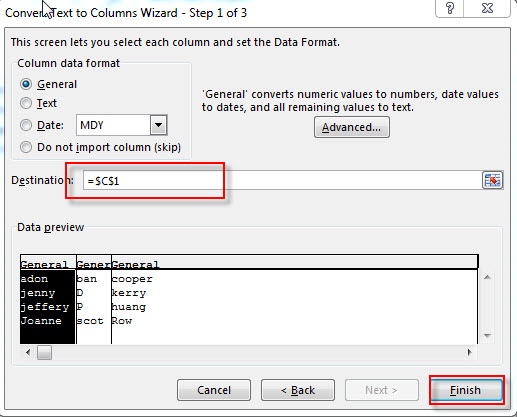5# you will see that all full names have been split into three columns.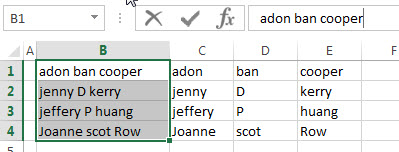6# select cells that contain middle name, then click Data-> Sort A to Z command, choose “Expand the selection” radio button, then click “sort” button.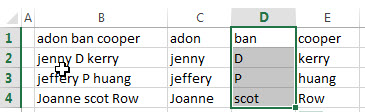### Related Posts

• Split full name to first and last name
You can create another formula based on the RIGHT function, the LEN function, the FIND function and the SUBSTITUTE function to extract the last name……
• Sort Name by Last Name in Excel
Assuming that you have a list of names in your worksheet and you would like to alphabetize by last name. You can create an excel formula based on the RIGHT function, the LEN function, the FIND function and the SUBSTITUTE function.
• Get first word from text string
If you want to extract the first word from a text string in a cell, you can use a combination of the IF function, the ISERR function, the LEFT function and the FIND function to create a complex excel formula..…
• Get last word from text string
If you want to get the last word from a text string, you can create an excel formula based on the RIGHT function, the LEN function, the FIND function and the SUBSTITUTE function..…
• Extract nth word from text string
If you want to extract the nth word from a text string in a single cell, you can create an excel formula based on the TRIM function, the MID function, the SUBSTITUTE function, the REPT function and the LEN function..…

### Related Functions

• Excel IF function
The Excel IF function perform a logical test to return one value if the condition is TRUE and return another value if the condition is FALSE. The IF function is a build-in function in Microsoft Excel and it is categorized as a Logical Function.The syntax of the IF function is as below:= IF (condition, [true_value], [false_value])….
• Excel ISERR function
The Excel ISERR function returns TRUE if the value is any error value except #N/A.The syntax of the ISERR function is as below:= ISERR (value)…
• Excel FIND function
The Excel FIND function returns the position of the first text string (sub string) within another text string.The syntax of the FIND function is as below:= FIND(find_text, within_text,[start_num])…
• Excel Substitute function
The Excel SUBSTITUTE function replaces a new text string for an old text string in a text string.The syntax of the SUBSTITUTE function is as below:= SUBSTITUTE  (text, old_text, new_text,[instance_num])….
• Excel MID function
The Excel MID function returns a substring from a text string at the position that you specify.The syntax of the MID function is as below:= MID (text, start_num, num_chars)….